# Lookup up price for product or service in Excel

In this article, we will learn how to LOOKUP date with last value in list in Excel.

Scenario:

We have a long table entry of Products and its service charges. We need to find the exact price for the product with its service charges. VLOOKUP function calculates your total cost for the product & its service charges.

Here we will be using the LOOKUP function. Let's first get some knowledge about the function.

LOOKUP function finds the estimated value in the range and returns the value from the range or its corresponding value.

Syntax:

= LOOKUP ( lookup_value, lookup_vector, [result_vector]

lookup_value: value to look up for
lookup_vector: array where the function looks for the lookup_value.
[result vector]:[optional] if the return array is different from the lookup array.
How to solve the problem.
Here we will be using the LOOKUP function multiple times, so as to get all required values. We will be using the below formula
Generic Formula:

= LOOKUP ( product , products_array , price_array )

product : lookup value
products_array : Array to look for value
price_array : Aray to return price value
Example
Here we have a products price list and Service cost list. We need to find the total cost for the required fields.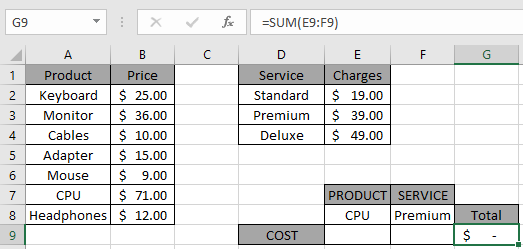So First we find the price for the CPU product
Formula:

= LOOKUP ( E8 , product , price )

product : named range used for the array (A2:A8)
price : named range used for the array (B2:B8)
Explanation:

• LOOKUP function looks for the Product (CPU) in the product array.
• The function finds the value and looks for its price in the corresponding price array.
• The function finds the price for the product and returns the price in the cell.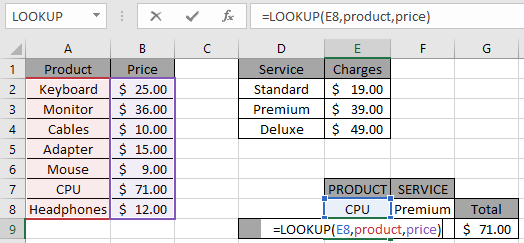Press Enter to get the results.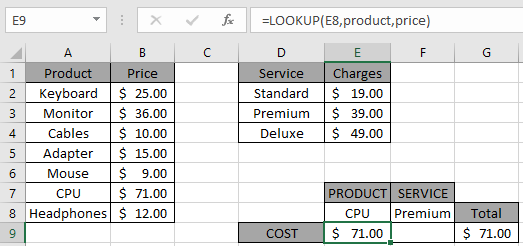As you can see the Price for product CPU is \$ 71.00.

Now To get the total cost. We also need to get the cost desired service charges.

Secondly we need to find the price for the service charges (premium).

Formula: = LOOKUP ( F8 , service , charges )

service : named range used for the array (D2:D4)

charges : named range used for the array (E2:E4)

Explanation:

• LOOKUP function looks for the Service (premium) in the service array.
• The function finds the value and looks for its charges in the corresponding charges array.
• The function finds the cost for the service (premium) and returns its charges in the cell.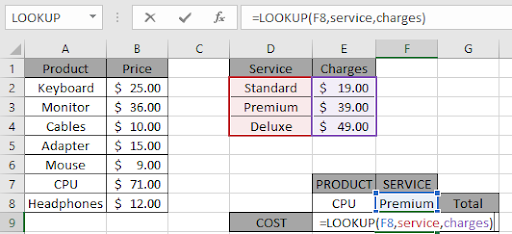Press Enter to get the results.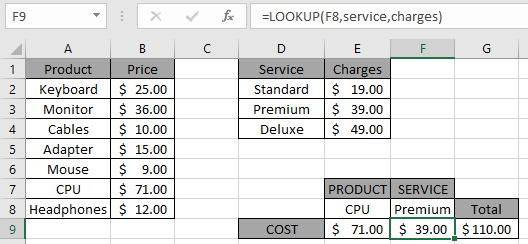The function returns the cost for both product and its service.

As you can see, the SUM function returns the SUM as required in the G9 cell. You can customize this LOOKUP Excel function as required.

Here we have observational notes for using the formula.

Notes:

1. The formula returns #NA error if no match is found in the array.
2. The formula finds the cost of the product and service by looking up the desired value.
3. You can use the VLOOKUP function instead of LOOKUP function.

Hope you understood how to Lookup up price for product or service in Excel. Explore more articles on lookup value here. Please feel free to state your queries below in the comment box. We will certainly help you.

Related Articles

Use INDEX and MATCH to Lookup Value : INDEX & MATCH function to look up value as required.

SUM range with INDEX in Excel : Use INDEX function to find the SUM of the values as required.

How to use the SUM function in Excel : Find the SUM of numbers using the SUM function explained with example.

How to use the INDEX function in Excel : Find the INDEX of array using the INDEX function explained with example.

How to use the MATCH function in Excel : Find the MATCH in the array using the INDEX value inside MATCH function explained with example.

How to use LOOKUP function in Excel : Find the lookup value in the array using the LOOKUP function explained with example.

How to use the VLOOKUP function in Excel : Find the lookup value in the array using the VLOOKUP function explained with example.

How to use the HLOOKUP function in Excel : Find the lookup value in the array using the HLOOKUP function explained with example.

Popular Articles

50 Excel Shortcut to Increase Your Productivity

Edit a dropdown list

Absolute reference in Excel

If with conditional formatting

If with wildcards

Vlookup by date

Join first and last name in excel

Terms and Conditions of use

The applications/code on this site are distributed as is and without warranties or liability. In no event shall the owner of the copyrights, or the authors of the applications/code be liable for any loss of profit, any problems or any damage resulting from the use or evaluation of the applications/code.